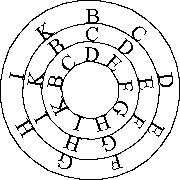4. The Fourth FigureThe fourth figure is made of three circles, two of which can be moved in order to multiply  cameras so that many solutions to questions can be found. This will be clear as the circles are moved, to anyone who diligently inquires into this, in the conclusions drawn through the scientific mixture of the principles and rules. And from this figure, the whole Table is derived, and each camera has twenty modes of response. This figure is general to the point of comprehending all the other figures and the whole practice of this science is implicitly contained in it. Each and every camera of this fourth figure contains three letters constituted according to the order of the three circles, so that B. of the largest circle is above C. of the second circle, and C. of the second circle above D. of the smallest circle, so as to form the first camera B.C.D., and likewise with the remaining cameras as the circles are moved in alphabetical sequence until 84 cameras have been drawn from this universal figure.